# NCERT Exemplar Class 10 Maths Solutions for Chapter 5 - Arithmetic Progressions

## NCERT Exemplar Solutions Class 10 Maths Chapter 5 – Free PDF Download

NCERT Exemplar Class 10 Maths Chapter 5 Arithmetic Progressions is provided here in PDF format so that students can download it. They can practice these questions to prepare for CBSE exams. These materials are prepared by our subject experts keeping into consideration the CBSE Syllabus (2021-2022). Students can download the exemplar problems with solutions and use them at their convenience to learn about the topics and also find answers to all the questions given in the chapter. Furthermore, students can use this as a reference tool to have a complete revision of the entire chapter before the exams.

The topic of arithmetic progression can sometimes be confusing for students. However, in Class 10, this topic has been broadly discussed in Chapter 5 Maths textbook. The chapter introduces students a lot of core concepts like an nth term of an AP, common difference, and more. There are also suitable examples provided to help students know the difference as well as the right method to find the sum of arithmetic progressions. Thus to make this chapter easy and fun, free NCERT Exemplar for arithmetic progressions is available here in the form of PDF for students. Click here to get exemplars for all chapters.

The topics covered in this material are related to:

• Arithmetic Progression – finding the first term and common difference
• Finding the nth term of AP
• Finding the sum of first n terms of AP
• Finding the sum of all terms of the AP
• Students can have a glimpse of Class 10 Maths Chapter 5 NCERT Exemplar PDF below.

## Download PDF of NCERT Exemplar Solutions for Class 10 Maths Chapter 5 Arithmetic Progression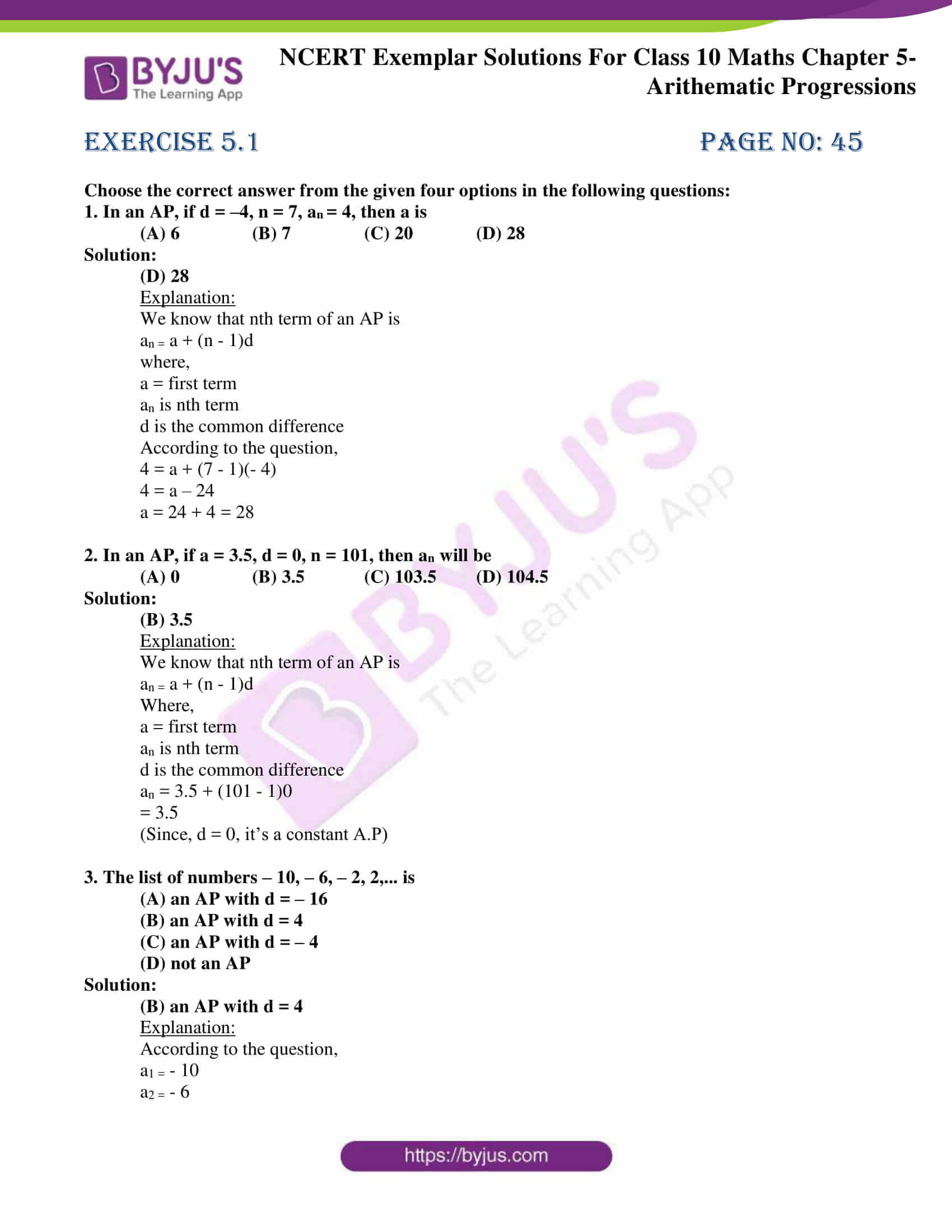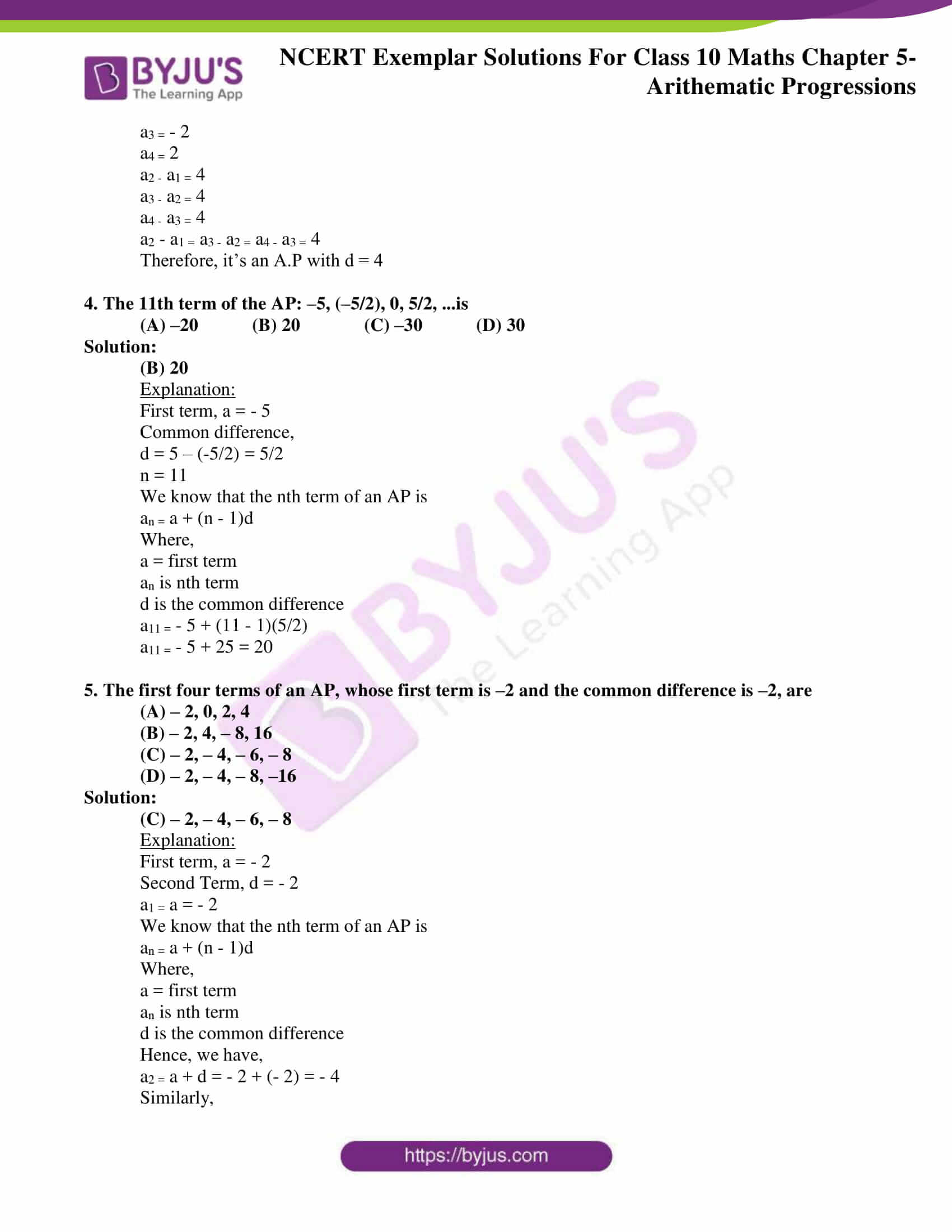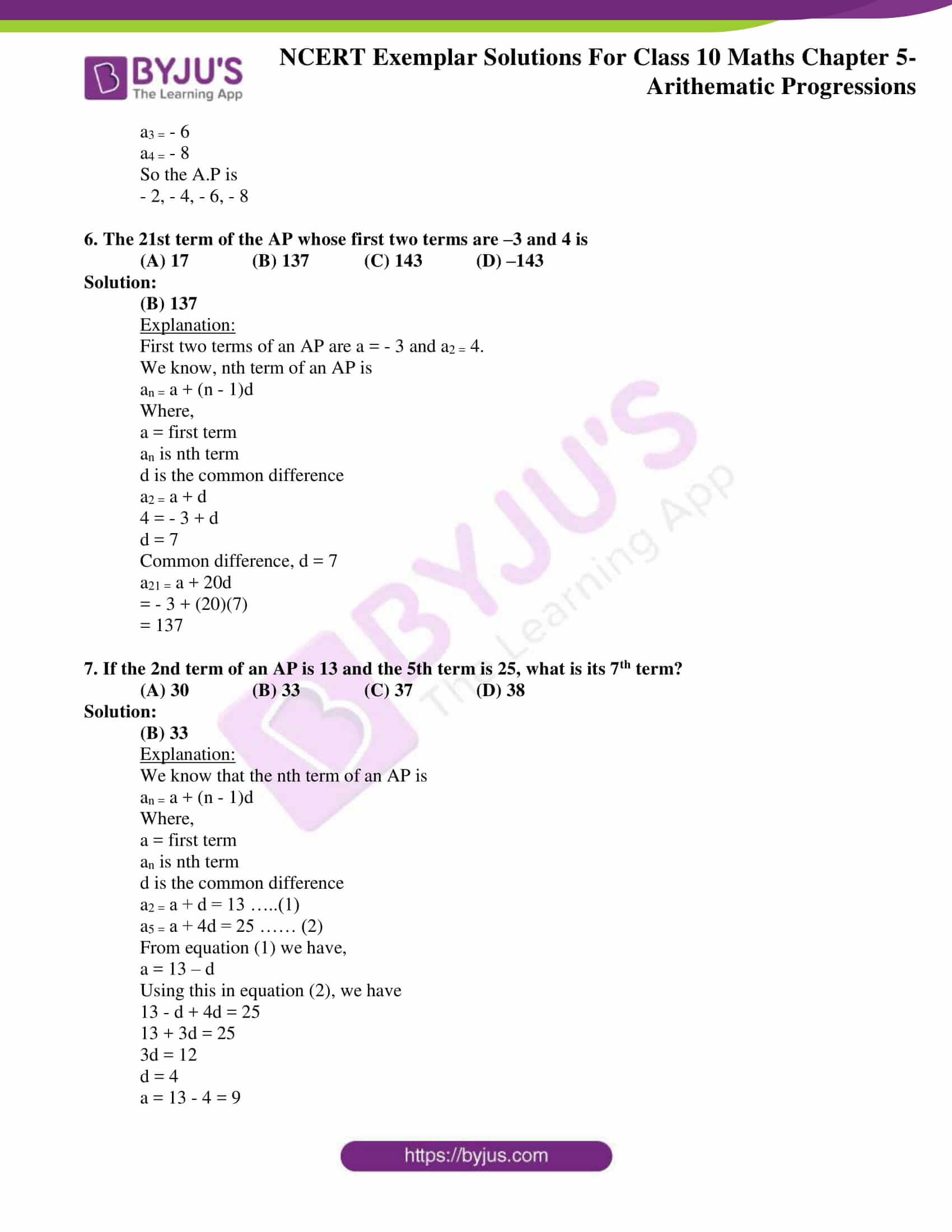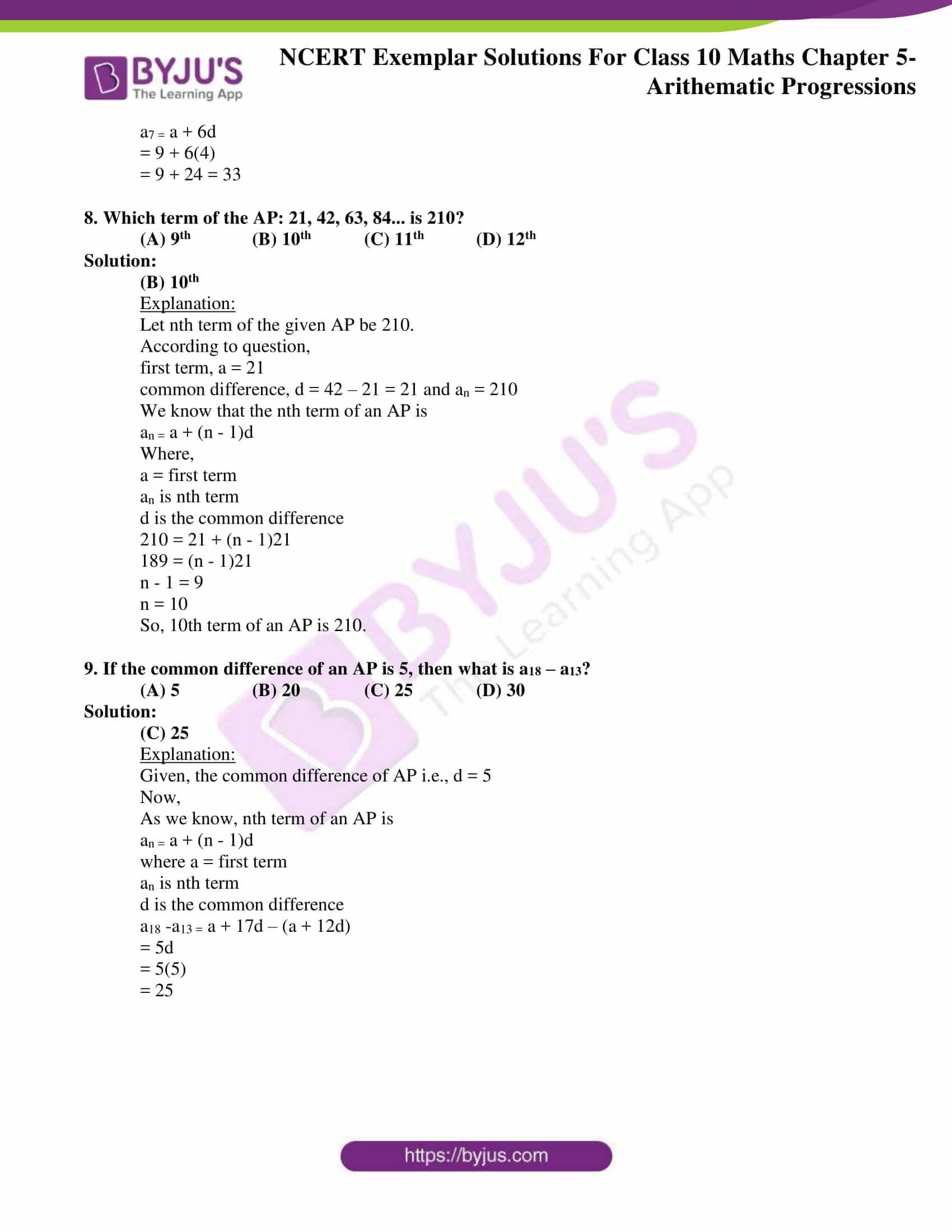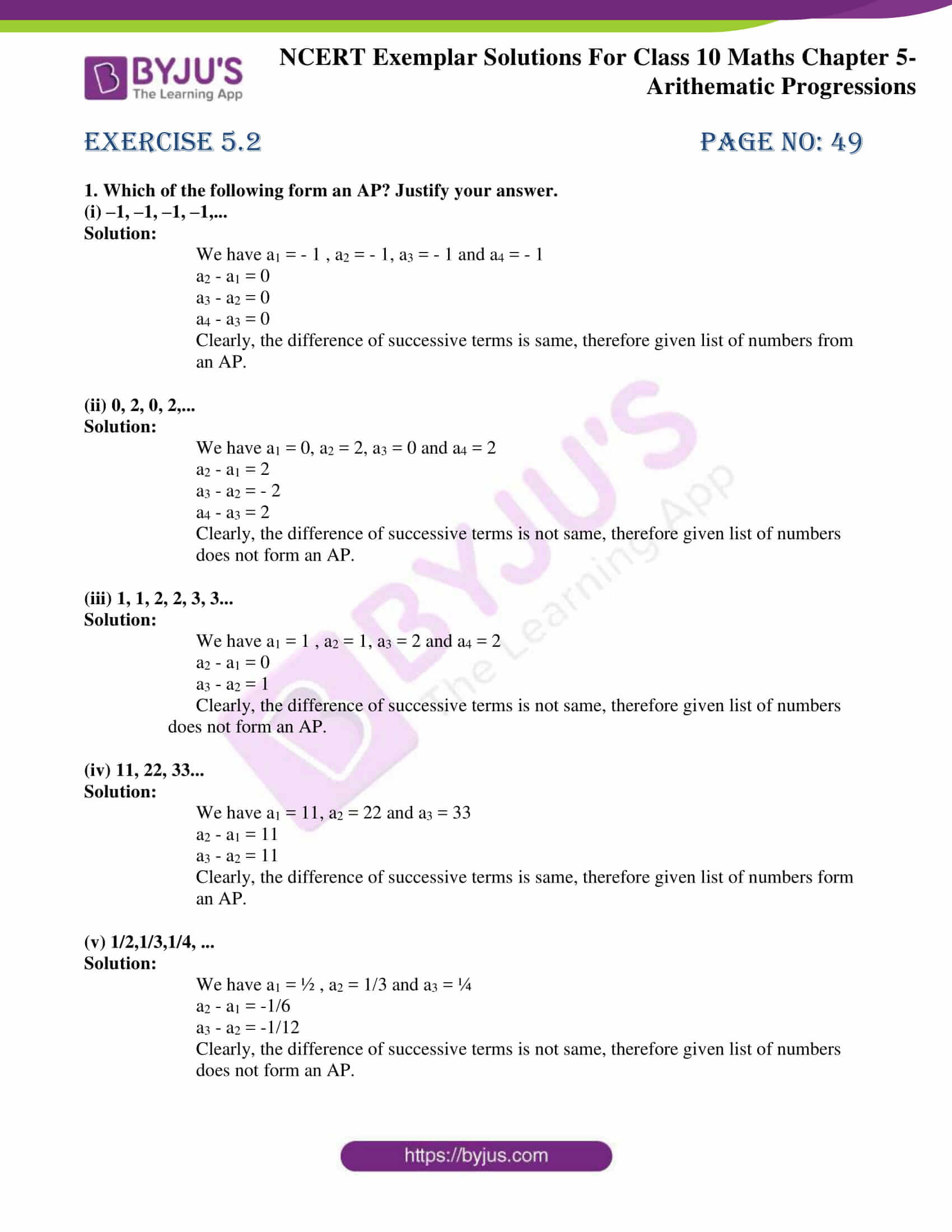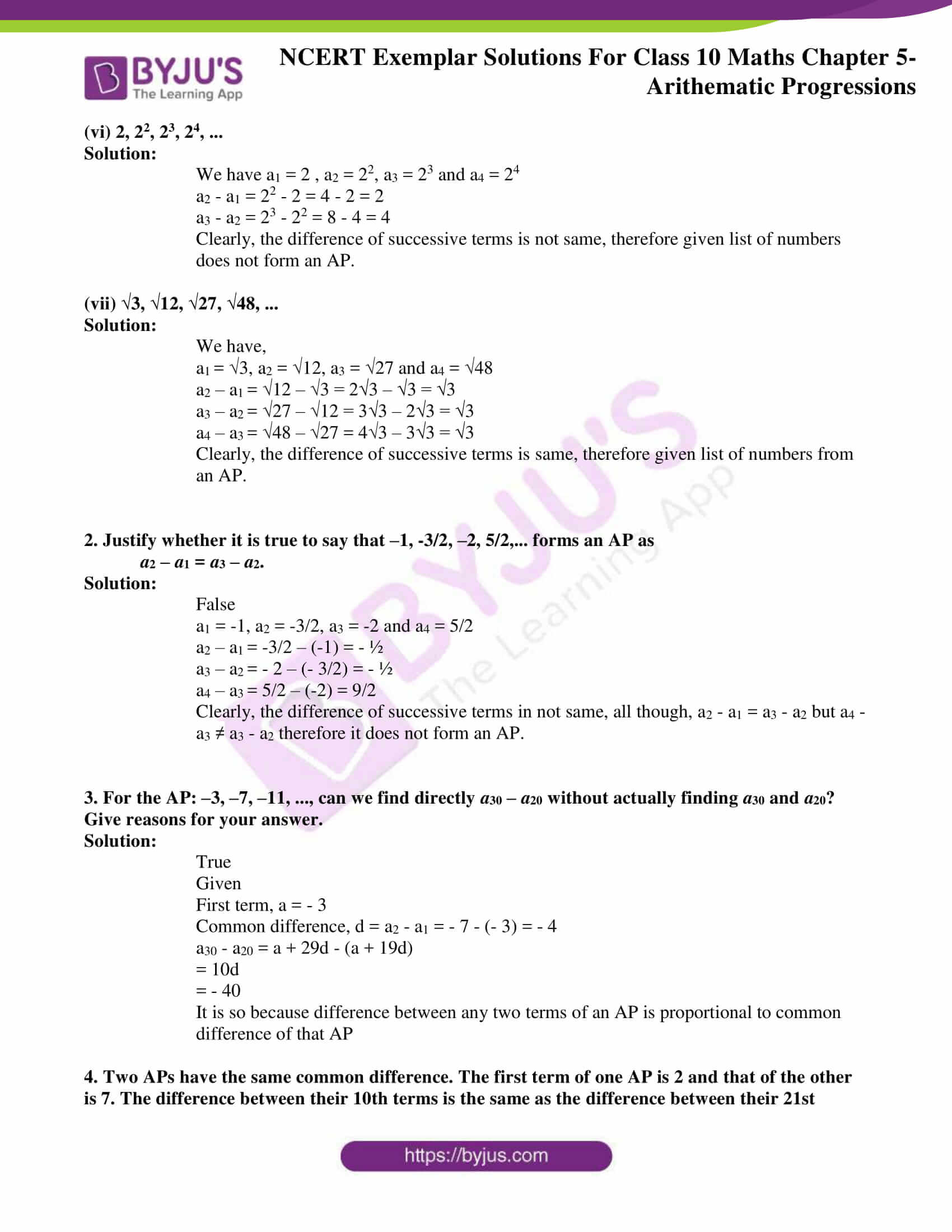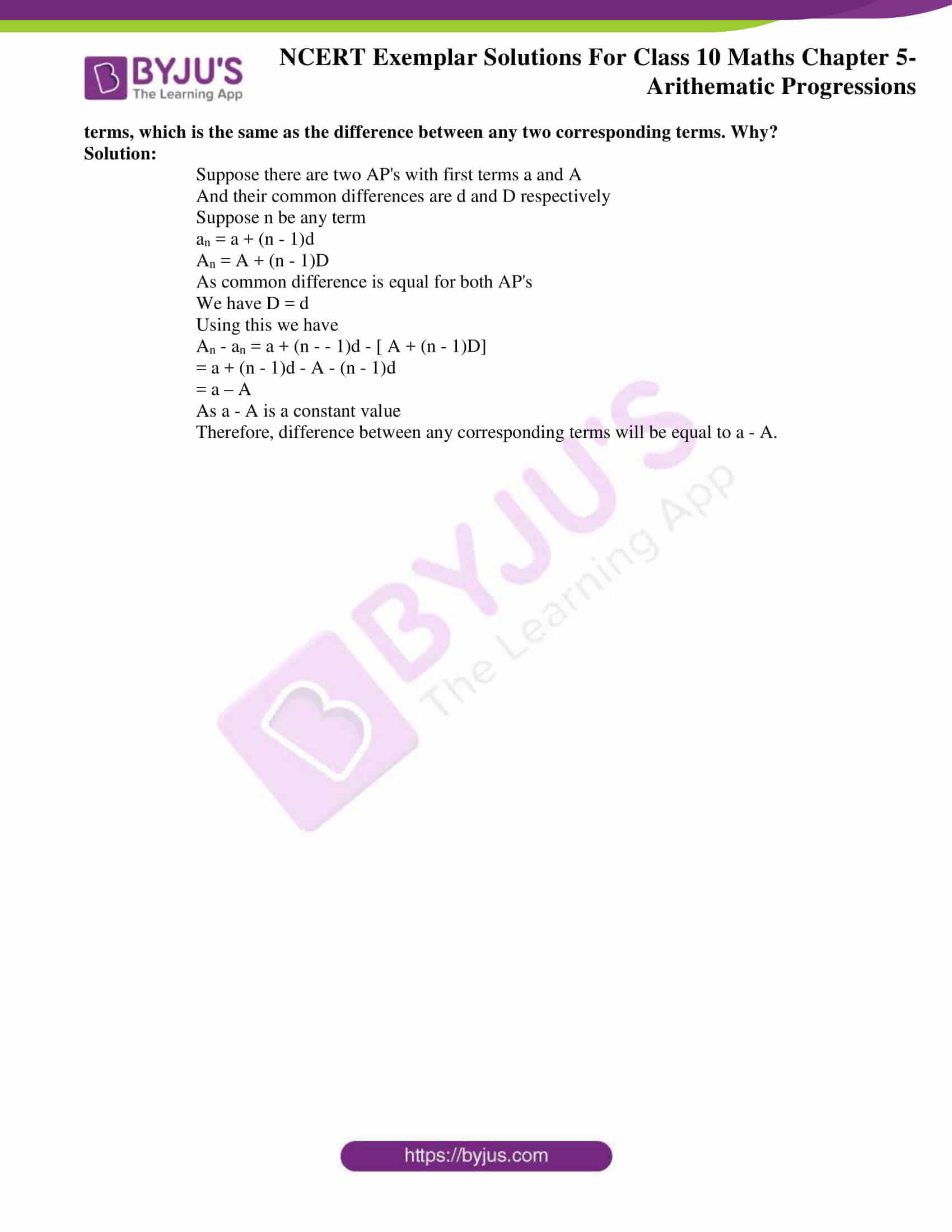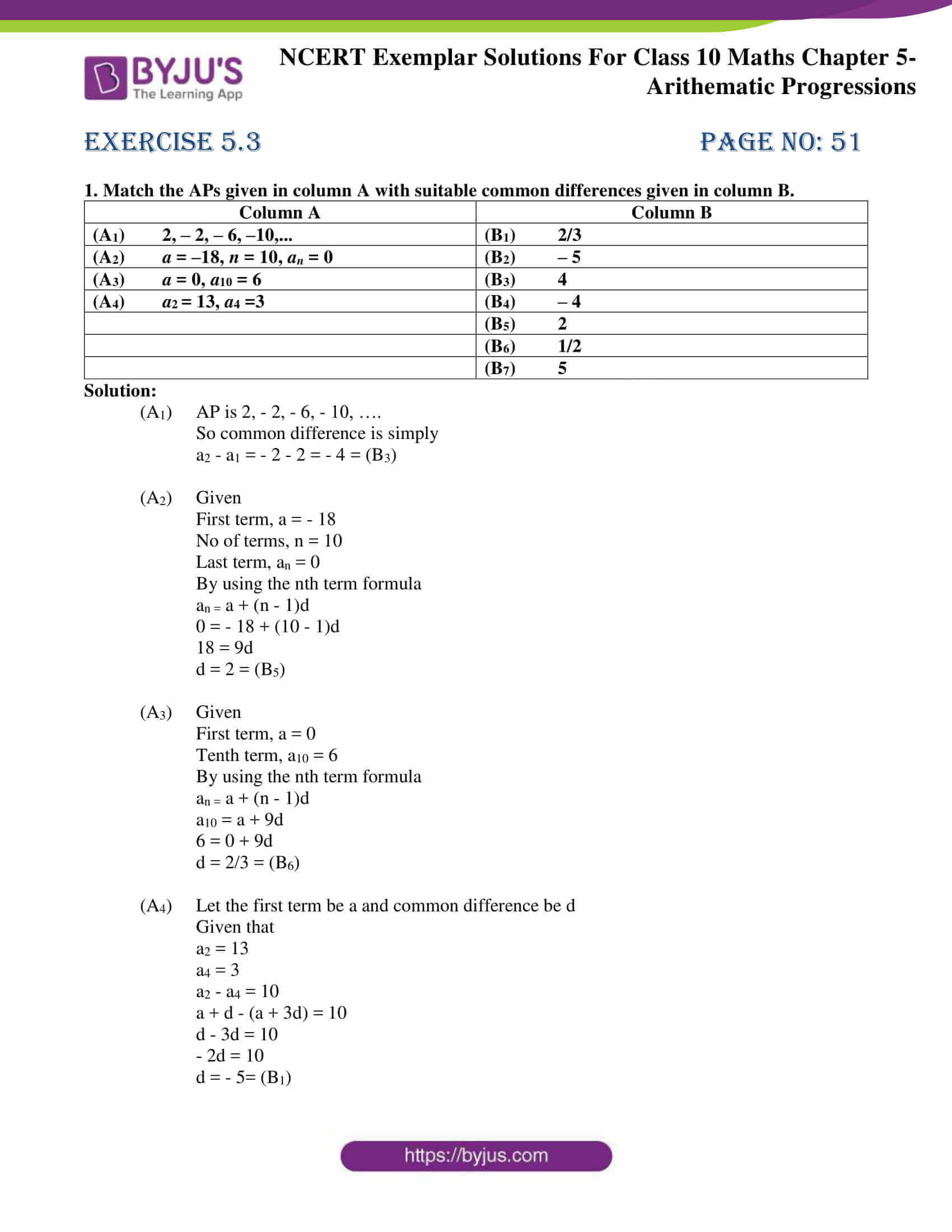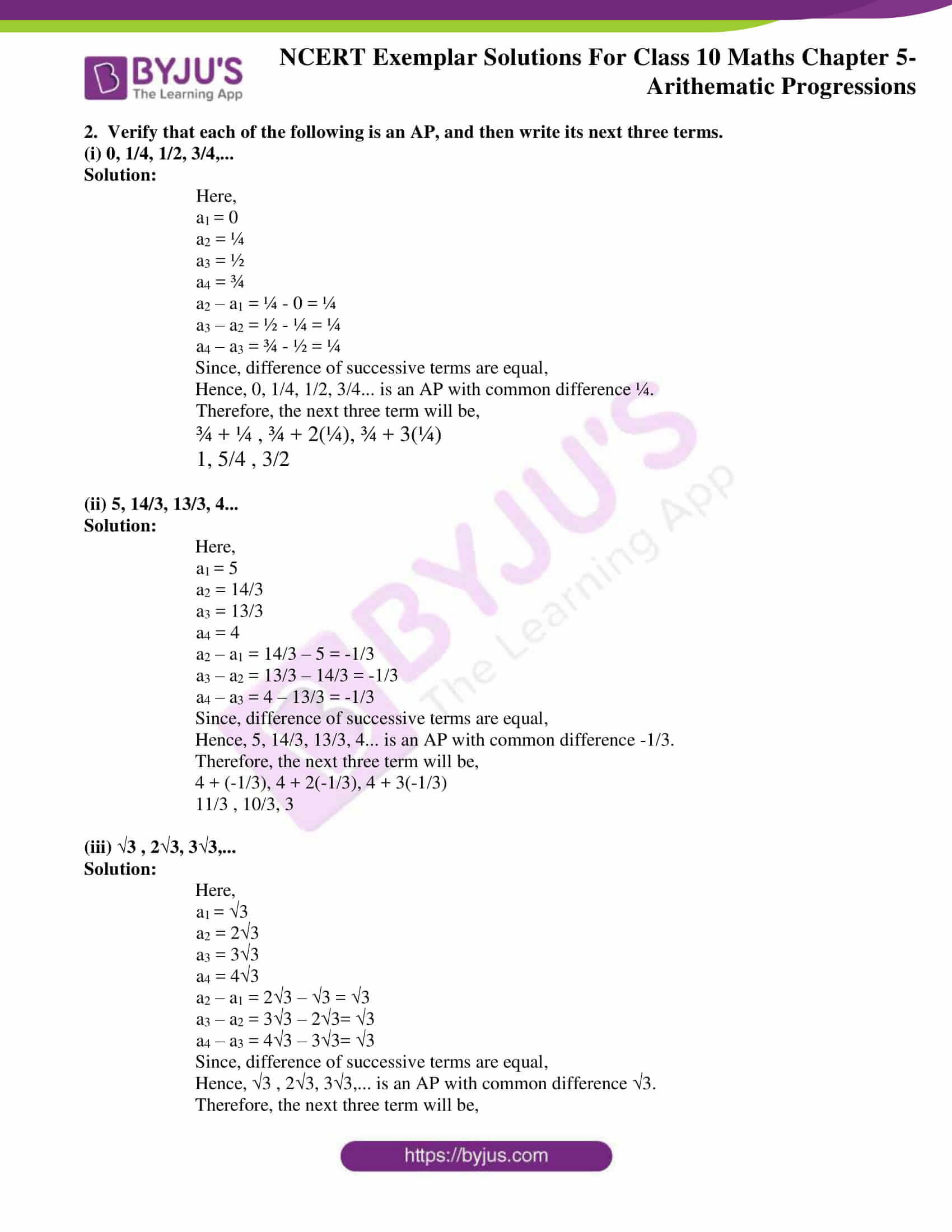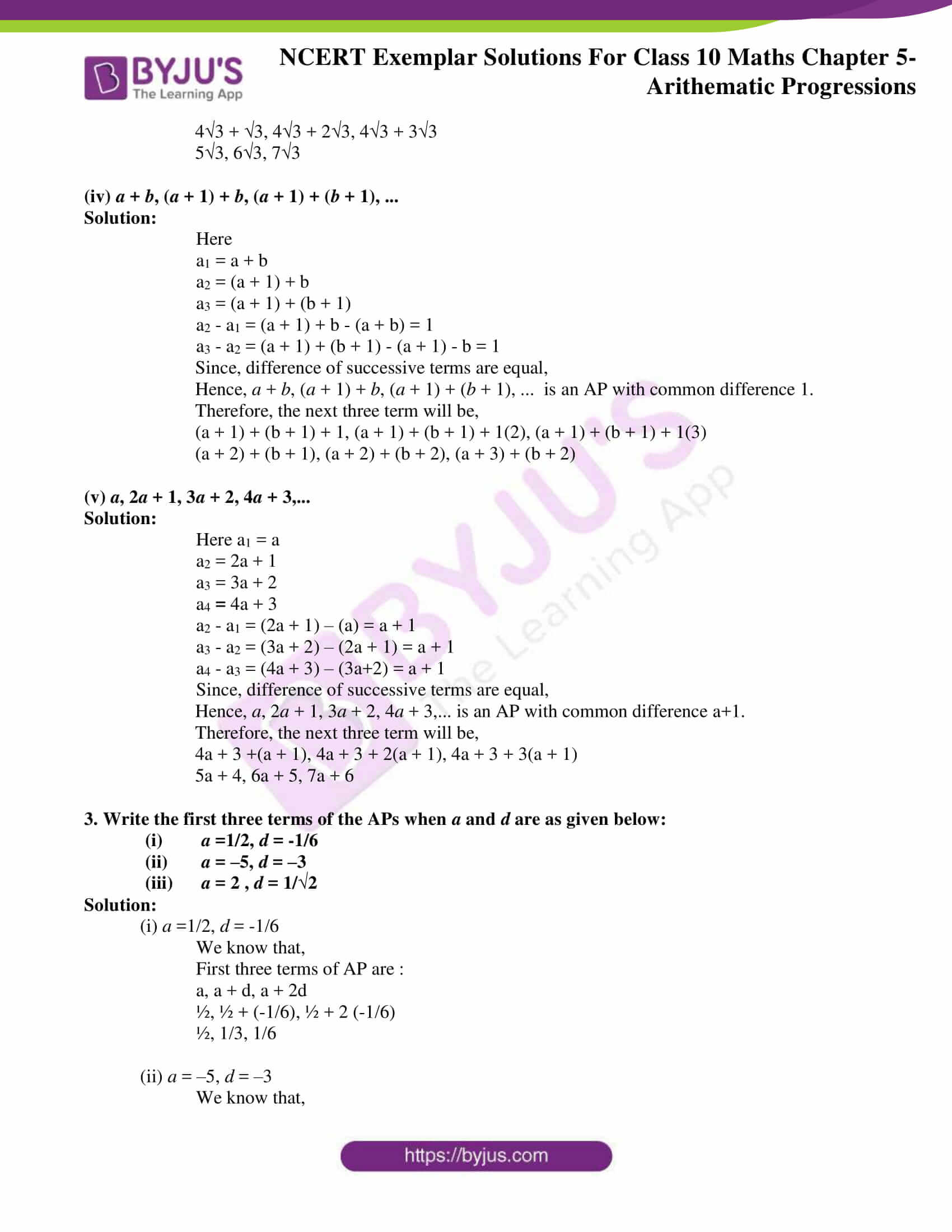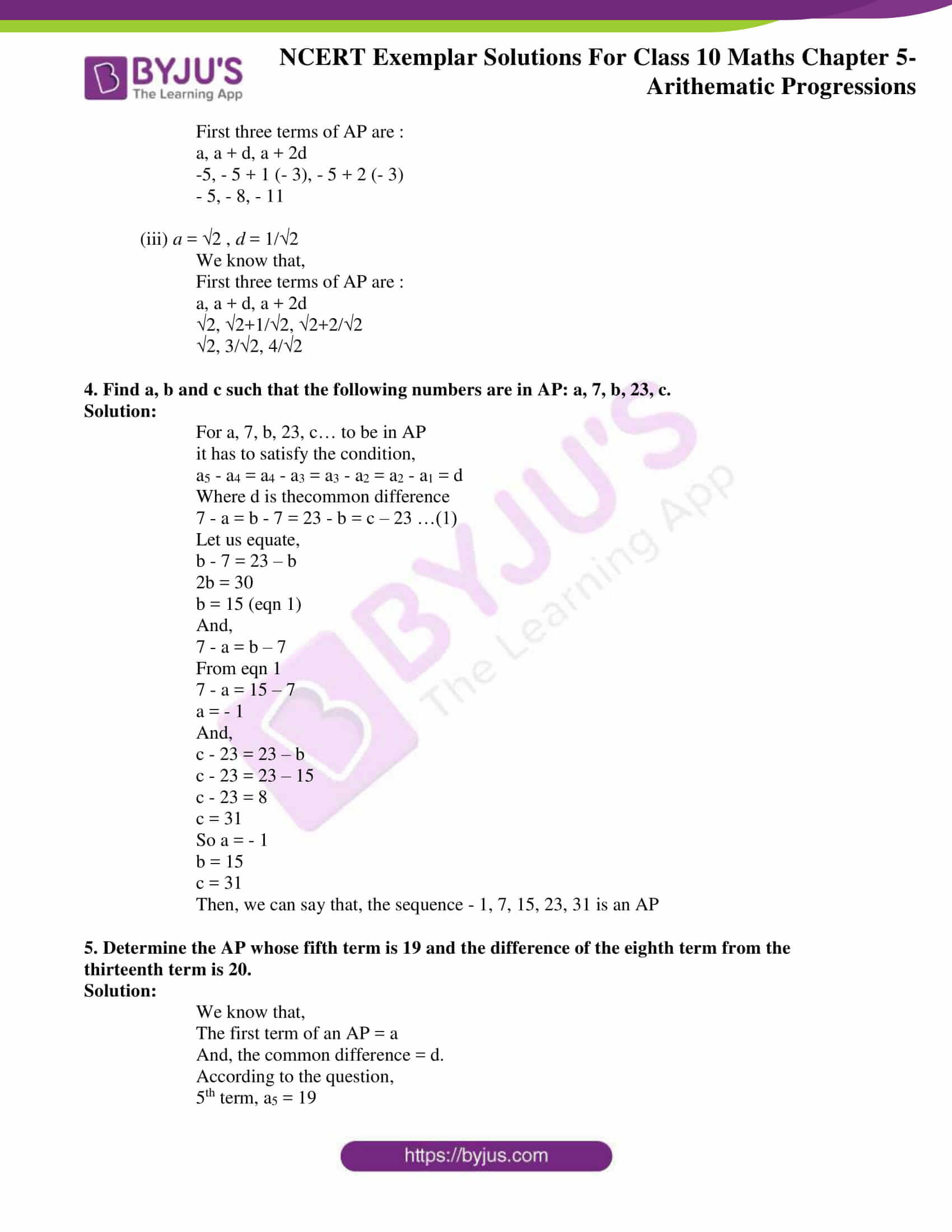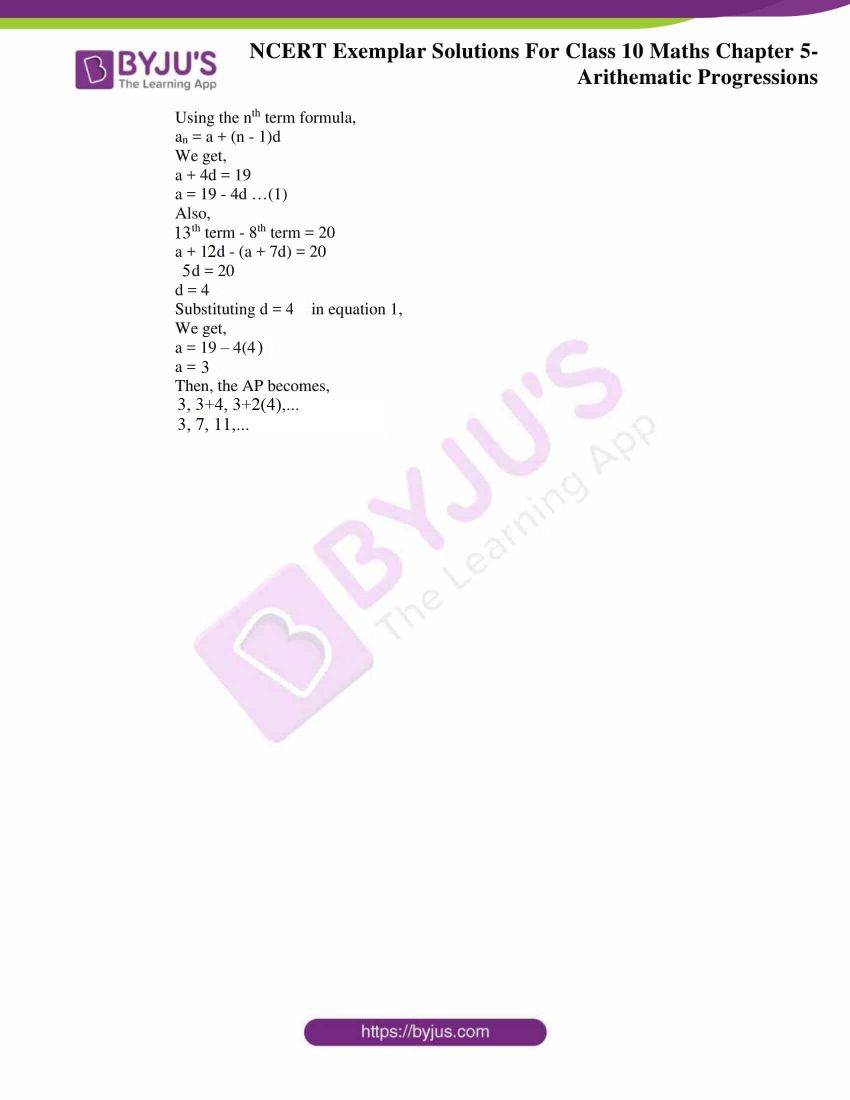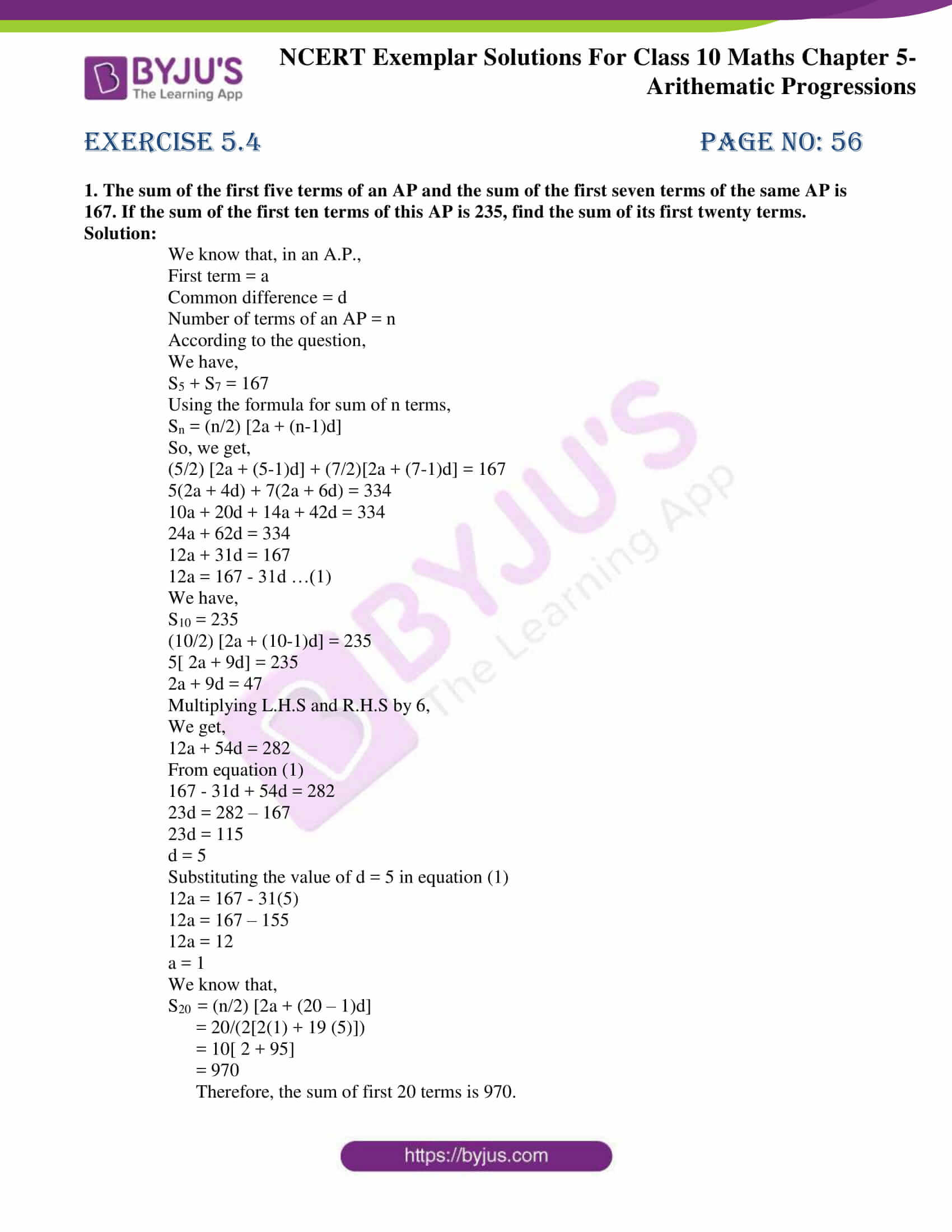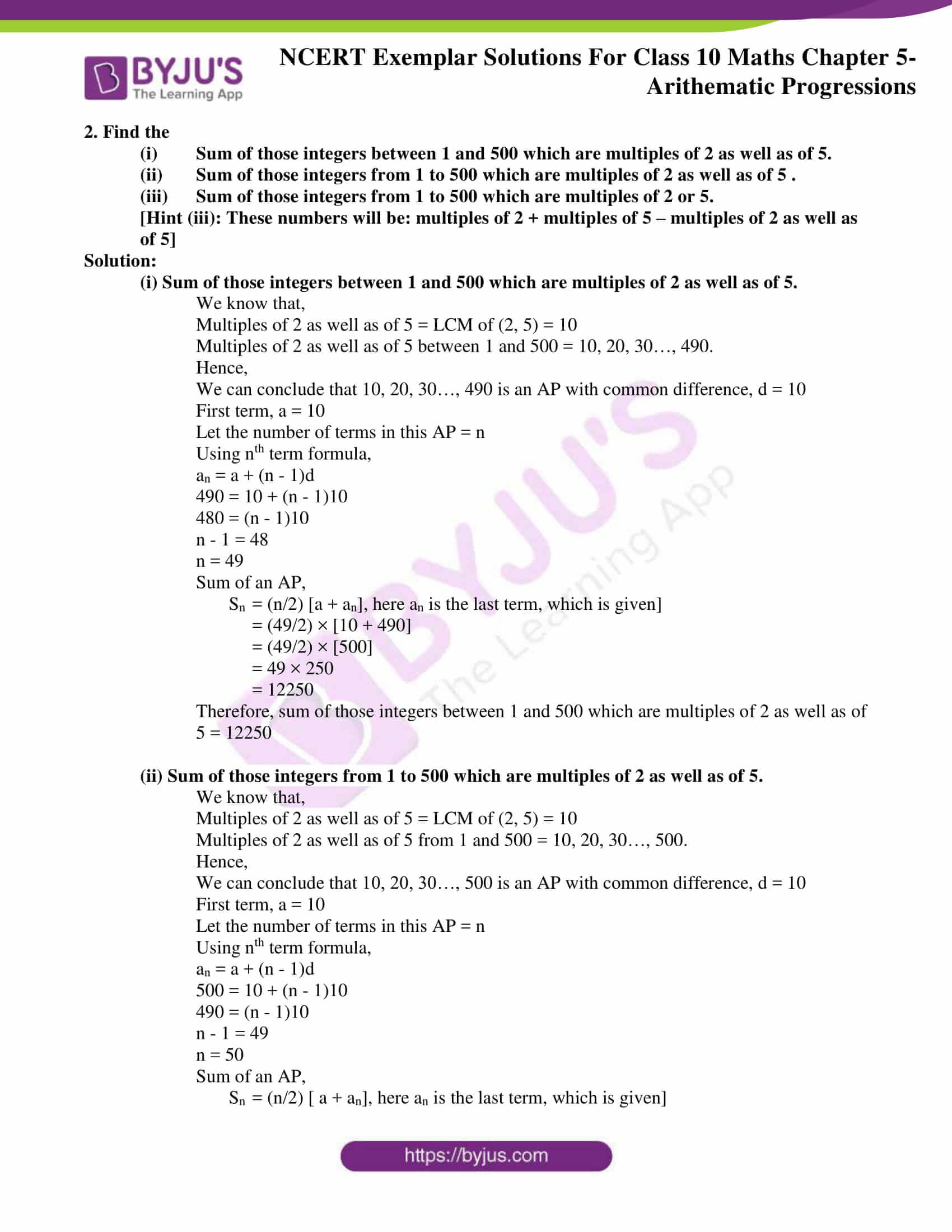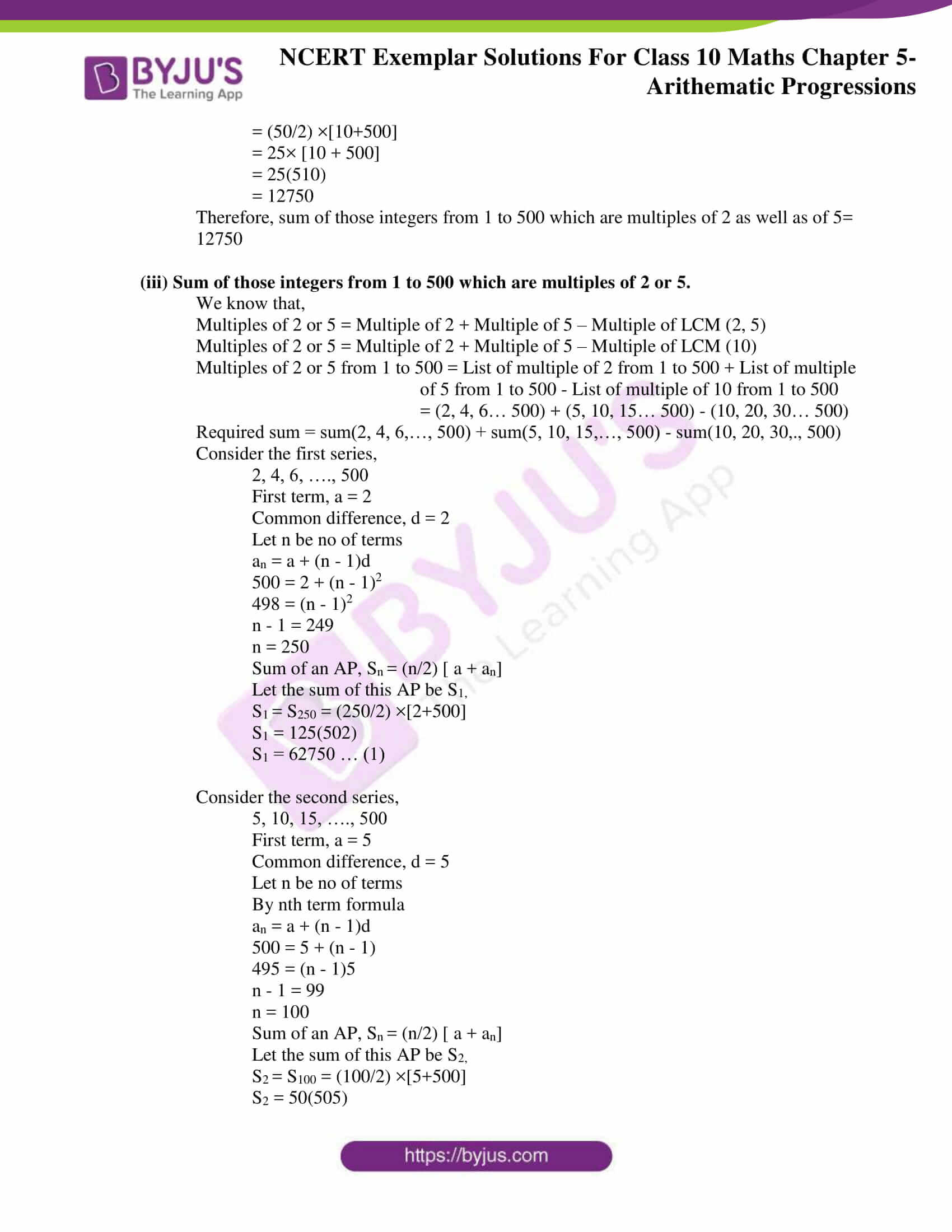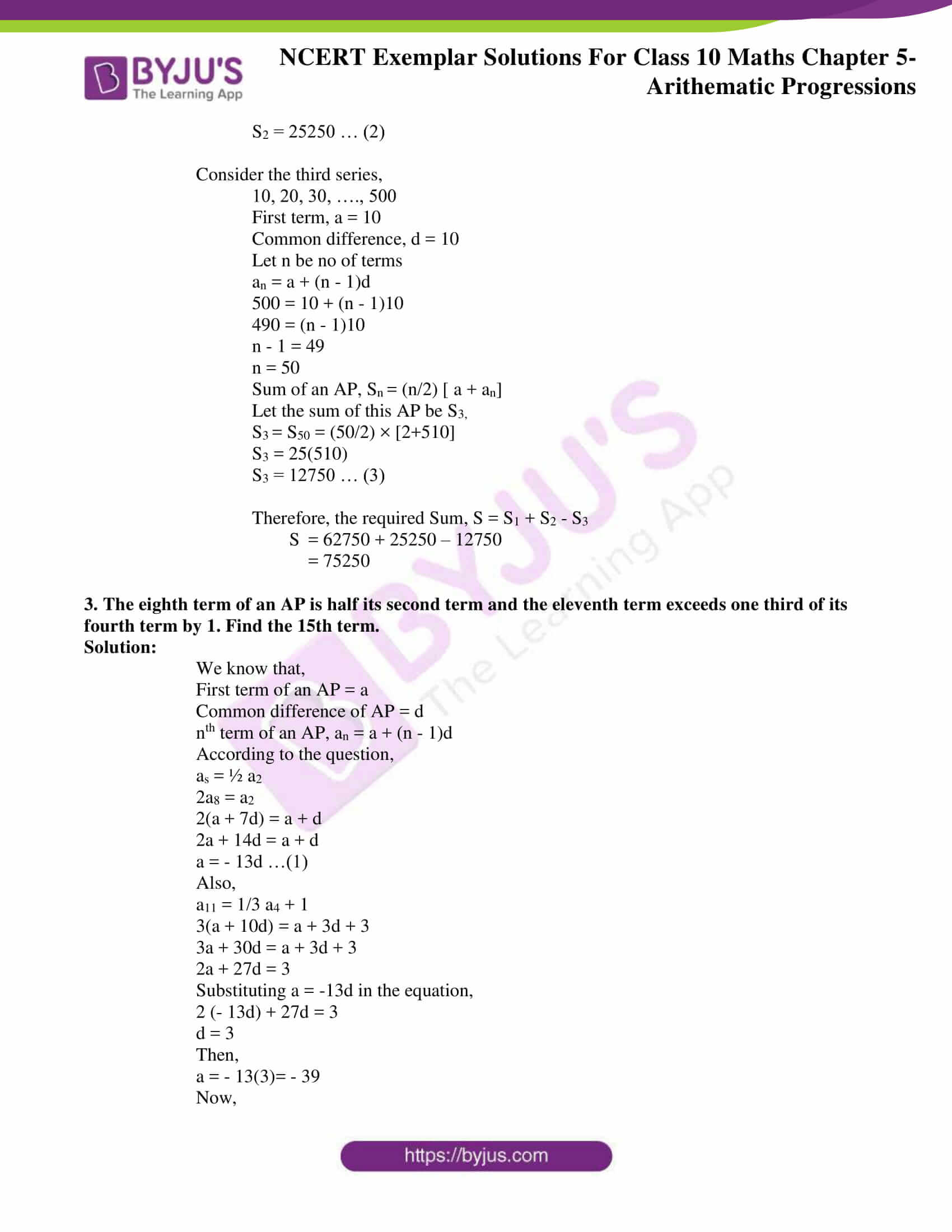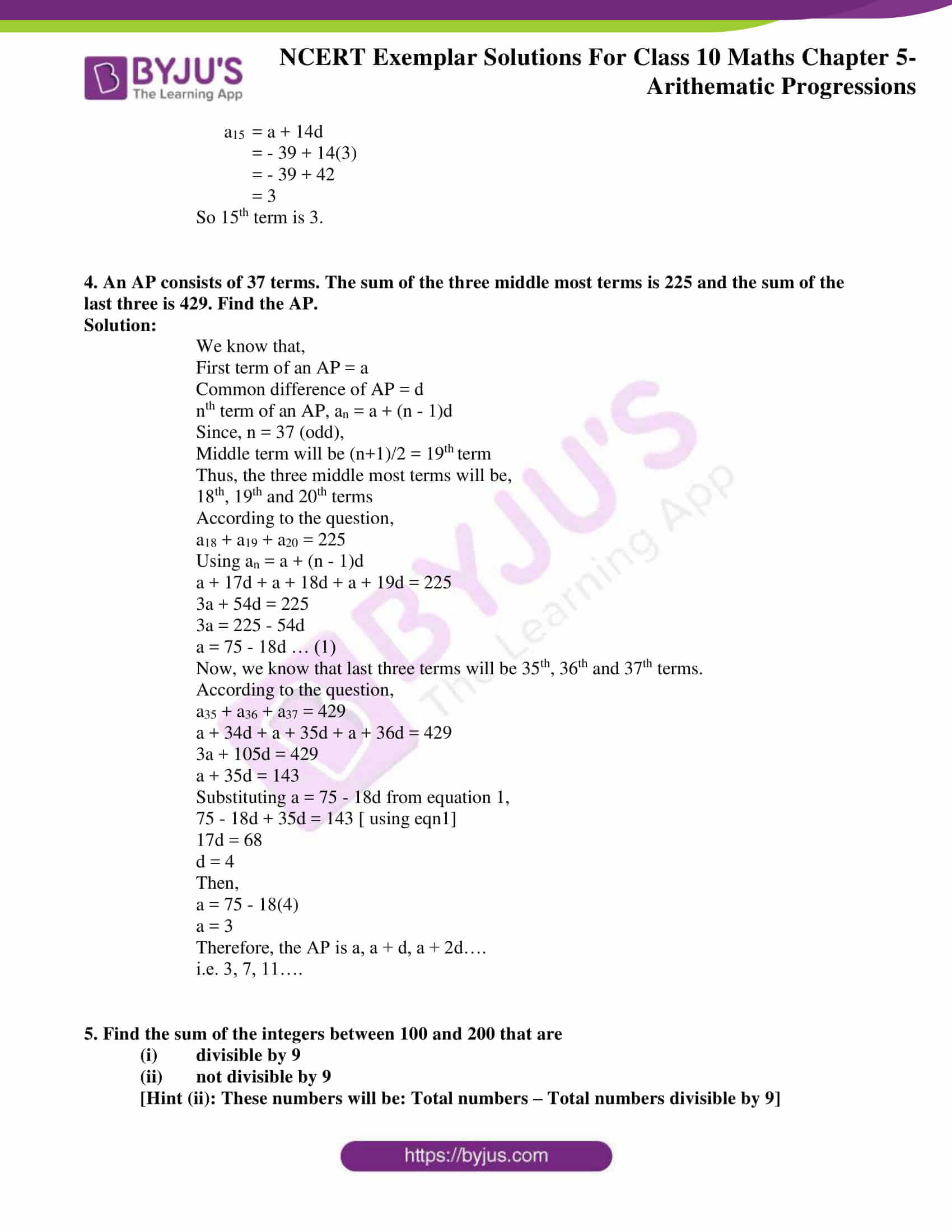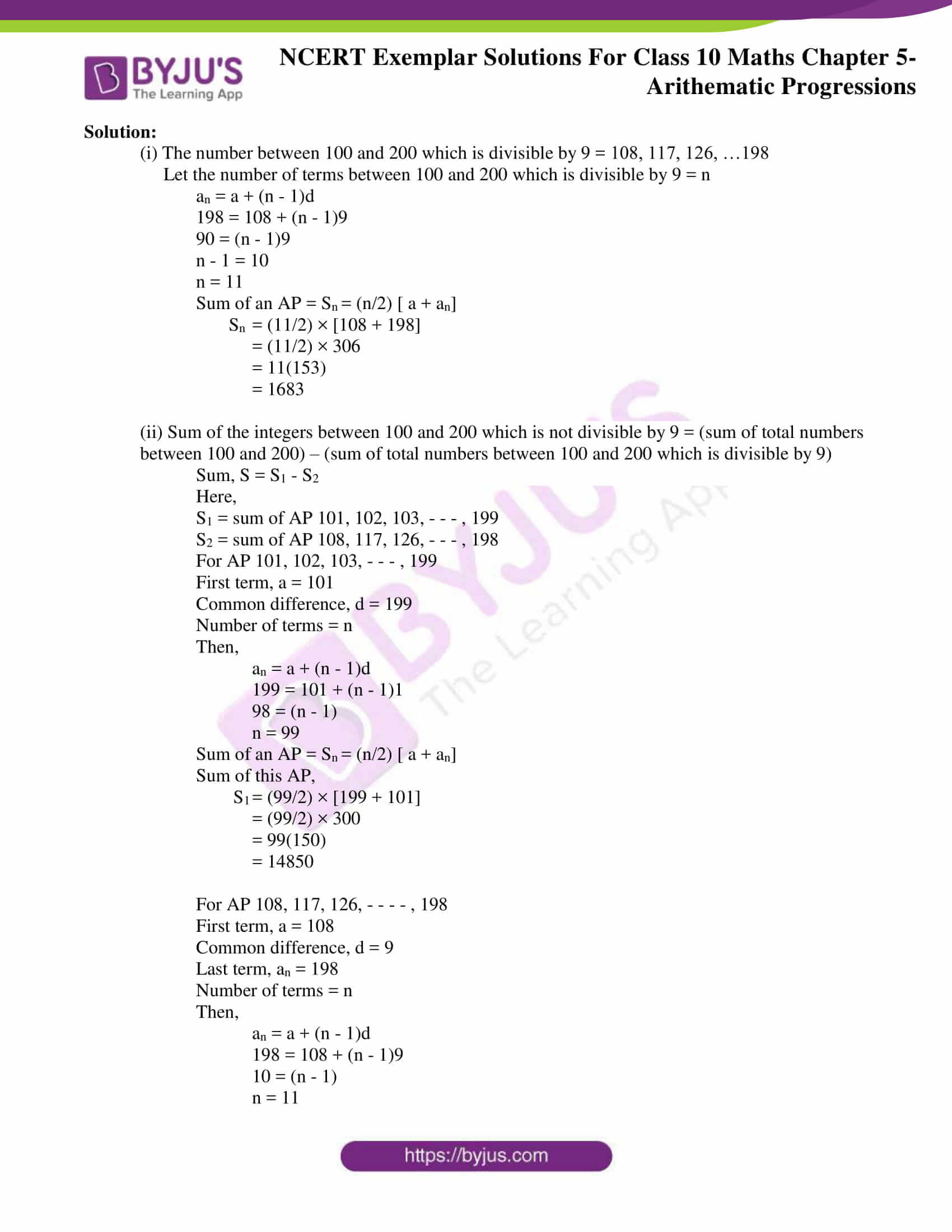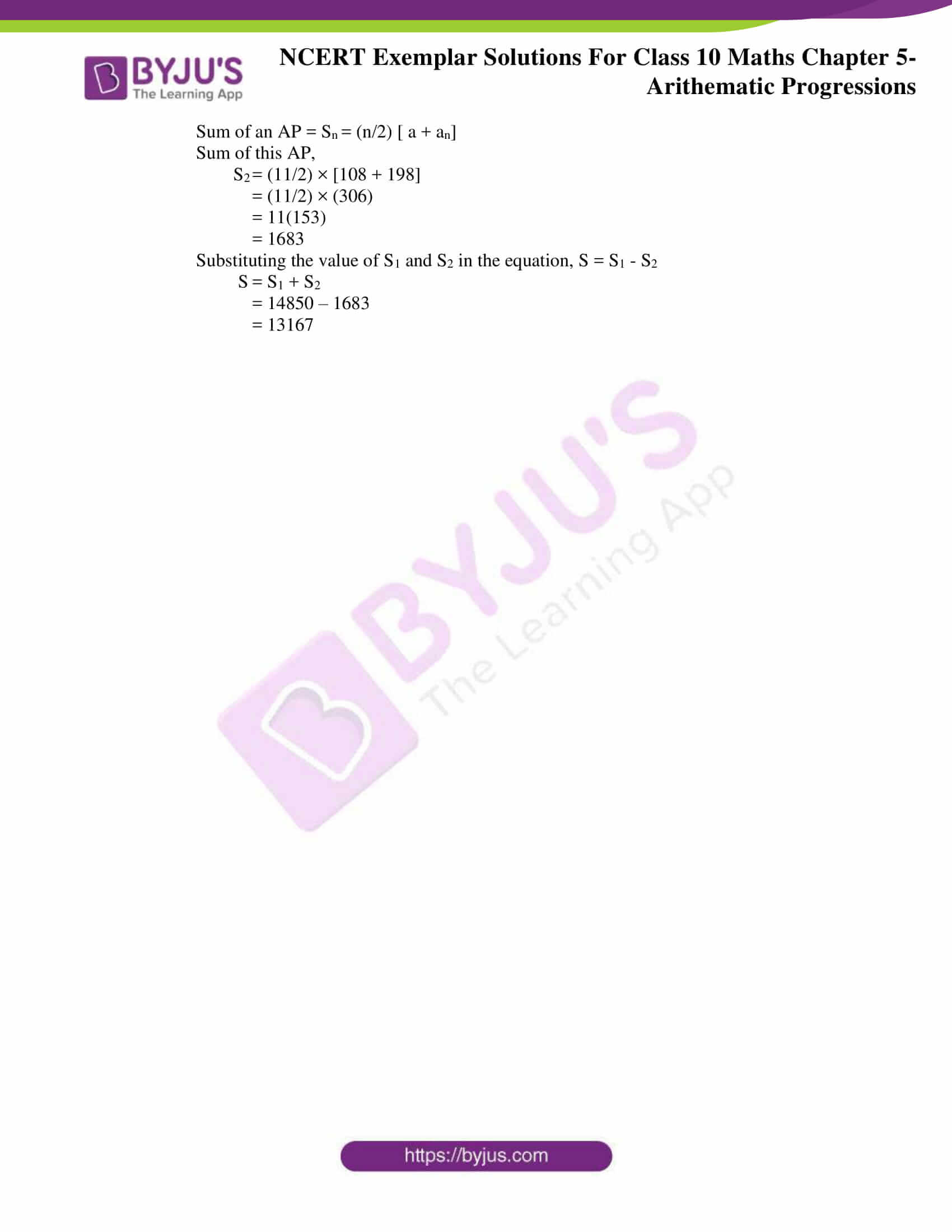## Exercise 5.1

Choose the correct answer from the given four options in the following questions:

1. In an AP, if d = –4, n = 7, an = 4, then a is

(A) 6 (B) 7 (C) 20 (D) 28

Solution:

(D) 28

Explanation:

We know that nth term of an AP is

an = a + (n – 1)d

where,

a = first term

an is nth term

d is the common difference

According to the question,

4 = a + (7 – 1)(- 4)

4 = a – 24

a = 24 + 4 = 28

2. In an AP, if a = 3.5, d = 0, n = 101, then an will be

(A) 0 (B) 3.5 (C) 103.5 (D) 104.5

Solution:

(B) 3.5

Explanation:

We know that nth term of an AP is

an = a + (n – 1)d

Where,

a = first term

an is nth term

d is the common difference

an = 3.5 + (101 – 1)0

= 3.5

(Since, d = 0, it’s a constant A.P)

3. The list of numbers – 10, – 6, – 2, 2,… is

(A) an AP with d = – 16

(B) an AP with d = 4

(C) an AP with d = – 4

(D) not an AP

Solution:

(B) an AP with d = 4

Explanation:

According to the question,

a1 = – 10

a2 = – 6

a3 = – 2

a4 = 2

a2 – a1 = 4

a3 – a2 = 4

a4 – a3 = 4

a2 – a1 = a3 – a2 = a4 – a3 = 4

Therefore, it’s an A.P with d = 4

4. The 11th term of the AP: –5, (–5/2), 0, 5/2, …is

(A) –20 (B) 20 (C) –30 (D) 30

Solution:

(B) 20

Explanation:

First term, a = – 5

Common difference,

d = 5 – (-5/2) = 5/2

n = 11

We know that the nth term of an AP is

an = a + (n – 1)d

Where,

a = first term

an is nth term

d is the common difference

a11 = – 5 + (11 – 1)(5/2)

a11 = – 5 + 25 = 20

5. The first four terms of an AP, whose first term is –2 and the common difference is –2, are

(A) – 2, 0, 2, 4

(B) – 2, 4, – 8, 16

(C) – 2, – 4, – 6, – 8

(D) – 2, – 4, – 8, –16

Solution:

(C) – 2, – 4, – 6, – 8

Explanation:

First term, a = – 2

Second Term, d = – 2

a1 = a = – 2

We know that the nth term of an AP is

an = a + (n – 1)d

Where,

a = first term

an is nth term

d is the common difference

Hence, we have,

a2 = a + d = – 2 + (- 2) = – 4

Similarly,

a3 = – 6

a4 = – 8

So the A.P is

– 2, – 4, – 6, – 8

6. The 21st term of the AP whose first two terms are –3 and 4 is

(A) 17 (B) 137 (C) 143 (D) –143

Solution:

(B) 137

Explanation:

First two terms of an AP are a = – 3 and a2 = 4.

We know, nth term of an AP is

an = a + (n – 1)d

Where,

a = first term

an is nth term

d is the common difference

a2 = a + d

4 = – 3 + d

d = 7

Common difference, d = 7

a21 = a + 20d

= – 3 + (20)(7)

= 137

7. If the 2nd term of an AP is 13 and the 5th term is 25, what is its 7th term?

(A) 30 (B) 33 (C) 37 (D) 38

Solution:

(B) 33

Explanation:

We know that the nth term of an AP is

an = a + (n – 1)d

Where,

a = first term

an is nth term

d is the common difference

a2 = a + d = 13 …..(1)

a5 = a + 4d = 25 …… (2)

From equation (1) we have,

a = 13 – d

Using this in equation (2), we have

13 – d + 4d = 25

13 + 3d = 25

3d = 12

d = 4

a = 13 – 4 = 9

a7 = a + 6d

= 9 + 6(4)

= 9 + 24 = 33

8. Which term of the AP: 21, 42, 63, 84… is 210?

(A) 9th (B) 10th (C) 11th (D) 12th

Solution:

(B) 10th

Explanation:

Let nth term of the given AP be 210.

According to question,

first term, a = 21

common difference, d = 42 – 21 = 21 and an = 210

We know that the nth term of an AP is

an = a + (n – 1)d

Where,

a = first term

an is nth term

d is the common difference

210 = 21 + (n – 1)21

189 = (n – 1)21

n – 1 = 9

n = 10

So, 10th term of an AP is 210.

9. If the common difference of an AP is 5, then what is a18 – a13?

(A) 5 (B) 20 (C) 25 (D) 30

Solution:

(C) 25

Explanation:

Given, the common difference of AP i.e., d = 5

Now,

As we know, nth term of an AP is

an = a + (n – 1)d

where a = first term

an is nth term

d is the common difference

a18 -a13 = a + 17d – (a + 12d)

= 5d

= 5(5)

= 25

## Exercise 5.2

(i) –1, –1, –1, –1,…

Solution:

We have a1 = – 1 , a2 = – 1, a3 = – 1 and a4 = – 1

a2 – a1 = 0

a3 – a2 = 0

a4 – a3 = 0

Clearly, the difference of successive terms is same, therefore given list of numbers from an AP.

(ii) 0, 2, 0, 2,…

Solution:

We have a1 = 0, a2 = 2, a3 = 0 and a4 = 2

a2 – a1 = 2

a3 – a2 = – 2

a4 – a3 = 2

Clearly, the difference of successive terms is not same, therefore given list of numbers does not form an AP.

(iii) 1, 1, 2, 2, 3, 3…

Solution:

We have a1 = 1 , a2 = 1, a3 = 2 and a4 = 2

a2 – a1 = 0

a3 – a2 = 1

Clearly, the difference of successive terms is not same, therefore given list of numbers does not form an AP.

(iv) 11, 22, 33…

Solution:

We have a1 = 11, a2 = 22 and a3 = 33

a2 – a1 = 11

a3 – a2 = 11

Clearly, the difference of successive terms is same, therefore given list of numbers form an AP.

(v) 1/2,1/3,1/4, …

Solution:

We have a1 = ½ , a2 = 1/3 and a3 = ¼

a2 – a1 = -1/6

a3 – a2 = -1/12

Clearly, the difference of successive terms is not same, therefore given list of numbers does not form an AP.

(vi) 2, 22, 23, 24, …

Solution:

We have a1 = 2 , a2 = 22, a3 = 23 and a4 = 24

a2 – a1 = 22 – 2 = 4 – 2 = 2

a3 – a2 = 23 – 22 = 8 – 4 = 4

Clearly, the difference of successive terms is not same, therefore given list of numbers does not form an AP.

(vii) √3, √12, √27, √48, …

Solution:

We have,

a1 = √3, a2 = √12, a3 = √27 and a4 = √48

a2 – a1 = √12 – √3 = 2√3 – √3 = √3

a3 – a2 = √27 – √12 = 3√3 – 2√3 = √3

a4 – a3 = √48 – √27 = 4√3 – 3√3 = √3

Clearly, the difference of successive terms is same, therefore given list of numbers from an AP.

2. Justify whether it is true to say that –1, -3/2, –2, 5/2,… forms an AP as

a2a1 = a3a2.

Solution:

False

a1 = -1, a2 = -3/2, a3 = -2 and a4 = 5/2

a2 – a1 = -3/2 – (-1) = – ½

a3 – a2 = – 2 – (- 3/2) = – ½

a4 – a3 = 5/2 – (-2) = 9/2

Clearly, the difference of successive terms in not same, all though, a2 – a1 = a3 – a2 but a4 – a3 ≠ a3 – a2 therefore it does not form an AP.

3. For the AP: –3, –7, –11, …, can we find directly a30a20 without actually finding a30 and a20? Give reasons for your answer.

Solution:

True

Given

First term, a = – 3

Common difference, d = a2 – a1 = – 7 – (- 3) = – 4

a30 – a20 = a + 29d – (a + 19d)

= 10d

= – 40

It is so because difference between any two terms of an AP is proportional to common difference of that AP

4. Two APs have the same common difference. The first term of one AP is 2 and that of the other is 7. The difference between their 10th terms is the same as the difference between their 21st terms, which is the same as the difference between any two corresponding terms. Why?

Solution:

Suppose there are two AP’s with first terms a and A

And their common differences are d and D respectively

Suppose n be any term

an = a + (n – 1)d

An = A + (n – 1)D

As common difference is equal for both AP’s

We have D = d

Using this we have

An – an = a + (n – – 1)d – [ A + (n – 1)D]

= a + (n – 1)d – A – (n – 1)d

= a – A

As a – A is a constant value

Therefore, difference between any corresponding terms will be equal to a – A.

## Exercise 5.3

1. Match the APs given in column A with suitable common differences given in column B.

 Column A Column B (A1) 2, – 2, – 6, –10,… (B1) 2/3 (A2) a = –18, n = 10, an = 0 (B2) – 5 (A3) a = 0, a10 = 6 (B3) 4 (A4) a2 = 13, a4 =3 (B4) – 4 (B5) 2 (B6) 1/2 (B7) 5

Solution:

(A1) AP is 2, – 2, – 6, – 10, ….

So common difference is simply

a2 – a1 = – 2 – 2 = – 4 = (B3)

(A2) Given

First term, a = – 18

No of terms, n = 10

Last term, an = 0

By using the nth term formula

an = a + (n – 1)d

0 = – 18 + (10 – 1)d

18 = 9d

d = 2 = (B5)

(A3) Given

First term, a = 0

Tenth term, a10 = 6

By using the nth term formula

an = a + (n – 1)d

a10 = a + 9d

6 = 0 + 9d

d = 2/3 = (B6)

(A4) Let the first term be a and common difference be d

Given that

a2 = 13

a4 = 3

a2 – a4 = 10

a + d – (a + 3d) = 10

d – 3d = 10

– 2d = 10

d = – 5= (B1)

2. Verify that each of the following is an AP, and then write its next three terms.

(i) 0, 1/4, 1/2, 3/4,…

Solution:

Here,

a1 = 0

a2 = ¼

a3 = ½

a4 = ¾

a2 – a1 = ¼ – 0 = ¼

a3 – a2 = ½ – ¼ = ¼

a4 – a3 = ¾ – ½ = ¼

Since, difference of successive terms are equal,

Hence, 0, 1/4, 1/2, 3/4… is an AP with common difference ¼.

Therefore, the next three term will be,

¾ + ¼ , ¾ + 2(¼), ¾ + 3(¼)

1, 5/4 , 3/2

(ii) 5, 14/3, 13/3, 4…

Solution:

Here,

a1 = 5

a2 = 14/3

a3 = 13/3

a4 = 4

a2 – a1 = 14/3 – 5 = -1/3

a3 – a2 = 13/3 – 14/3 = -1/3

a4 – a3 = 4 – 13/3 = -1/3

Since, difference of successive terms are equal,

Hence, 5, 14/3, 13/3, 4… is an AP with common difference -1/3.

Therefore, the next three term will be,

4 + (-1/3), 4 + 2(-1/3), 4 + 3(-1/3)

11/3 , 10/3, 3

(iii)3 , 23, 33,…

Solution:

Here,

a1 = √3

a2 = 2√3

a3 = 3√3

a4 = 4√3

a2 – a1 = 2√3 – √3 = √3

a3 – a2 = 3√3 – 2√3= √3

a4 – a3 = 4√3 – 3√3= √3

Since, difference of successive terms are equal,

Hence, √3 , 2√3, 3√3,… is an AP with common difference √3.

Therefore, the next three term will be,

4√3 + √3, 4√3 + 2√3, 4√3 + 3√3

5√3, 6√3, 7√3

(iv) a + b, (a + 1) + b, (a + 1) + (b + 1), …

Solution:

Here

a1 = a + b

a2 = (a + 1) + b

a3 = (a + 1) + (b + 1)

a2 – a1 = (a + 1) + b – (a + b) = 1

a3 – a2 = (a + 1) + (b + 1) – (a + 1) – b = 1

Since, difference of successive terms are equal,

Hence, a + b, (a + 1) + b, (a + 1) + (b + 1), … is an AP with common difference 1.

Therefore, the next three term will be,

(a + 1) + (b + 1) + 1, (a + 1) + (b + 1) + 1(2), (a + 1) + (b + 1) + 1(3)

(a + 2) + (b + 1), (a + 2) + (b + 2), (a + 3) + (b + 2)

(v) a, 2a + 1, 3a + 2, 4a + 3,…

Solution:

Here a1 = a

a2 = 2a + 1

a3 = 3a + 2

a4 = 4a + 3

a2 – a1 = (2a + 1) – (a) = a + 1

a3 – a2 = (3a + 2) – (2a + 1) = a + 1

a4 – a3 = (4a + 3) – (3a+2) = a + 1

Since, difference of successive terms are equal,

Hence, a, 2a + 1, 3a + 2, 4a + 3,… is an AP with common difference a+1.

Therefore, the next three term will be,

4a + 3 +(a + 1), 4a + 3 + 2(a + 1), 4a + 3 + 3(a + 1)

5a + 4, 6a + 5, 7a + 6

3. Write the first three terms of the APs when a and d are as given below:

1. a =1/2, d = -1/6
2. a = –5, d = –3
3. a = 2 , d = 1/2

Solution:

(i) a =1/2, d = -1/6

We know that,

First three terms of AP are :

a, a + d, a + 2d

½, ½ + (-1/6), ½ + 2 (-1/6)

½, 1/3, 1/6

(ii) a = –5, d = –3

We know that,

First three terms of AP are :

a, a + d, a + 2d

-5, – 5 + 1 (- 3), – 5 + 2 (- 3)

– 5, – 8, – 11

(iii) a = √2 , d = 1/√2

We know that,

First three terms of AP are :

a, a + d, a + 2d

√2, √2+1/√2, √2+2/√2

√2, 3/√2, 4/√2

4. Find a, b and c such that the following numbers are in AP: a, 7, b, 23, c.

Solution:

For a, 7, b, 23, c… to be in AP

it has to satisfy the condition,

a5 – a4 = a4 – a3 = a3 – a2 = a2 – a1 = d

Where d is thecommon difference

7 – a = b – 7 = 23 – b = c – 23 …(1)

Let us equate,

b – 7 = 23 – b

2b = 30

b = 15 (eqn 1)

And,

7 – a = b – 7

From eqn 1

7 – a = 15 – 7

a = – 1

And,

c – 23 = 23 – b

c – 23 = 23 – 15

c – 23 = 8

c = 31

So a = – 1

b = 15

c = 31

Then, we can say that, the sequence – 1, 7, 15, 23, 31 is an AP

5. Determine the AP whose fifth term is 19 and the difference of the eighth term from the thirteenth term is 20.

Solution:

We know that,

The first term of an AP = a

And, the common difference = d.

According to the question,

5th term, a5 = 19

Using the nth term formula,

an = a + (n – 1)d

We get,

a + 4d = 19

a = 19 – 4d …(1)

Also,

13th term – 8th term = 20

a + 12d – (a + 7d) = 20

5d = 20

d = 4

Substituting d = 4 in equation 1,

We get,

a = 19 – 4(4)

a = 3

Then, the AP becomes,

3, 3 + 4 , 3 + 2(4),…

3, 7, 11,…

## Exercise 5.4

1. The sum of the first five terms of an AP and the sum of the first seven terms of the same AP is 167. If the sum of the first ten terms of this AP is 235, find the sum of its first twenty terms.

Solution:

We know that, in an A.P.,

First term = a

Common difference = d

Number of terms of an AP = n

According to the question,

We have,

S5 + S7 = 167

Using the formula for sum of n terms,

Sn = (n/2) [2a + (n-1)d]

So, we get,

(5/2) [2a + (5-1)d] + (7/2)[2a + (7-1)d] = 167

5(2a + 4d) + 7(2a + 6d) = 334

10a + 20d + 14a + 42d = 334

24a + 62d = 334

12a + 31d = 167

12a = 167 – 31d …(1)

We have,

S10 = 235

(10/2) [2a + (10-1)d] = 235

5[ 2a + 9d] = 235

2a + 9d = 47

Multiplying L.H.S and R.H.S by 6,

We get,

12a + 54d = 282

From equation (1)

167 – 31d + 54d = 282

23d = 282 – 167

23d = 115

d = 5

Substituting the value of d = 5 in equation (1)

12a = 167 – 31(5)

12a = 167 – 155

12a = 12

a = 1

We know that,

S20 = (n/2) [2a + (20 – 1)d]

= 20/(2[2(1) + 19 (5)])

= 10[ 2 + 95]

= 970

Therefore, the sum of first 20 terms is 970.

2. Find the

(i) Sum of those integers between 1 and 500 which are multiples of 2 as well as of 5.

(ii) Sum of those integers from 1 to 500 which are multiples of 2 as well as of 5 .

(iii) Sum of those integers from 1 to 500 which are multiples of 2 or 5.

[Hint (iii): These numbers will be: multiples of 2 + multiples of 5 – multiples of 2 as well as of 5]

Solution:

(i) Sum of those integers between 1 and 500 which are multiples of 2 as well as of 5.

We know that,

Multiples of 2 as well as of 5 = LCM of (2, 5) = 10

Multiples of 2 as well as of 5 between 1 and 500 = 10, 20, 30…, 490.

Hence,

We can conclude that 10, 20, 30…, 490 is an AP with common difference, d = 10

First term, a = 10

Let the number of terms in this AP = n

Using nth term formula,

an = a + (n – 1)d

490 = 10 + (n – 1)10

480 = (n – 1)10

n – 1 = 48

n = 49

Sum of an AP,

Sn = (n/2) [a + an], here an is the last term, which is given]

= (49/2) × [10 + 490]

= (49/2) × 

= 49 × 250

= 12250

Therefore, sum of those integers between 1 and 500 which are multiples of 2 as well as of 5 = 12250

(ii) Sum of those integers from 1 to 500 which are multiples of 2 as well as of 5.

We know that,

Multiples of 2 as well as of 5 = LCM of (2, 5) = 10

Multiples of 2 as well as of 5 from 1 and 500 = 10, 20, 30…, 500.

Hence,

We can conclude that 10, 20, 30…, 500 is an AP with common difference, d = 10

First term, a = 10

Let the number of terms in this AP = n

Using nth term formula,

an = a + (n – 1)d

500 = 10 + (n – 1)10

490 = (n – 1)10

n – 1 = 49

n = 50

Sum of an AP,

Sn = (n/2) [ a + an], here an is the last term, which is given]

= (50/2) ×[10+500]

= 25× [10 + 500]

= 25(510)

= 12750

Therefore, sum of those integers from 1 to 500 which are multiples of 2 as well as of 5= 12750

(iii) Sum of those integers from 1 to 500 which are multiples of 2 or 5.

We know that,

Multiples of 2 or 5 = Multiple of 2 + Multiple of 5 – Multiple of LCM (2, 5)

Multiples of 2 or 5 = Multiple of 2 + Multiple of 5 – Multiple of LCM (10)

Multiples of 2 or 5 from 1 to 500 = List of multiple of 2 from 1 to 500 + List of multiple

of 5 from 1 to 500 – List of multiple of 10 from 1 to 500

= (2, 4, 6… 500) + (5, 10, 15… 500) – (10, 20, 30… 500)

Required sum = sum(2, 4, 6,…, 500) + sum(5, 10, 15,…, 500) – sum(10, 20, 30,., 500)

Consider the first series,

2, 4, 6, …., 500

First term, a = 2

Common difference, d = 2

Let n be no of terms

an = a + (n – 1)d

500 = 2 + (n – 1)2

498 = (n – 1)2

n – 1 = 249

n = 250

Sum of an AP, Sn = (n/2) [ a + an]

Let the sum of this AP be S1,

S1 = S250 = (250/2) ×[2+500]

S1 = 125(502)

S1 = 62750 … (1)

Consider the second series,

5, 10, 15, …., 500

First term, a = 5

Common difference, d = 5

Let n be no of terms

By nth term formula

an = a + (n – 1)d

500 = 5 + (n – 1)

495 = (n – 1)5

n – 1 = 99

n = 100

Sum of an AP, Sn = (n/2) [ a + an]

Let the sum of this AP be S2,

S2 = S100 = (100/2) ×[5+500]

S2 = 50(505)

S2 = 25250 … (2)

Consider the third series,

10, 20, 30, …., 500

First term, a = 10

Common difference, d = 10

Let n be no of terms

an = a + (n – 1)d

500 = 10 + (n – 1)10

490 = (n – 1)10

n – 1 = 49

n = 50

Sum of an AP, Sn = (n/2) [ a + an]

Let the sum of this AP be S3,

S3 = S50 = (50/2) × [2+510]

S3 = 25(510)

S3 = 12750 … (3)

Therefore, the required Sum, S = S1 + S2 – S3

S = 62750 + 25250 – 12750

= 75250

3. The eighth term of an AP is half its second term and the eleventh term exceeds one third of its fourth term by 1. Find the 15th term.

Solution:

We know that,

First term of an AP = a

Common difference of AP = d

nth term of an AP, an = a + (n – 1)d

According to the question,

as = ½ a2

2a8 = a2

2(a + 7d) = a + d

2a + 14d = a + d

a = – 13d …(1)

Also,

a11 = 1/3 a4 + 1

3(a + 10d) = a + 3d + 3

3a + 30d = a + 3d + 3

2a + 27d = 3

Substituting a = -13d in the equation,

2 (- 13d) + 27d = 3

d = 3

Then,

a = – 13(3)= – 39

Now,

a15  = a + 14d

= – 39 + 14(3)

= – 39 + 42

= 3

So 15th term is 3.

4. An AP consists of 37 terms. The sum of the three middle most terms is 225 and the sum of the last three is 429. Find the AP.

Solution:

We know that,

First term of an AP = a

Common difference of AP = d

nth term of an AP, an = a + (n – 1)d

Since, n = 37 (odd),

Middle term will be (n+1)/2 = 19th term

Thus, the three middle most terms will be,

18th, 19th and 20th terms

According to the question,

a18 + a19 + a20 = 225

Using an = a + (n – 1)d

a + 17d + a + 18d + a + 19d = 225

3a + 54d = 225

3a = 225 – 54d

a = 75 – 18d … (1)

Now, we know that last three terms will be 35th, 36th and 37th terms.

According to the question,

a35 + a36 + a37 = 429

a + 34d + a + 35d + a + 36d = 429

3a + 105d = 429

a + 35d = 143

Substituting a = 75 – 18d from equation 1,

75 – 18d + 35d = 143 [ using eqn1]

17d = 68

d = 4

Then,

a = 75 – 18(4)

a = 3

Therefore, the AP is a, a + d, a + 2d….

i.e. 3, 7, 11….

5. Find the sum of the integers between 100 and 200 that are

(i) divisible by 9
(ii) not divisible by 9

[Hint (ii): These numbers will be: Total numbers – Total numbers divisible by 9]

Solution:

(i) The number between 100 and 200 which is divisible by 9 = 108, 117, 126, …198

Let the number of terms between 100 and 200 which is divisible by 9 = n

an = a + (n – 1)d

198 = 108 + (n – 1)9

90 = (n – 1)9

n – 1 = 10

n = 11

Sum of an AP = Sn = (n/2) [ a + an]

Sn = (11/2) × [108 + 198]

= (11/2) × 306

= 11(153)

= 1683

(ii) Sum of the integers between 100 and 200 which is not divisible by 9 = (sum of total numbers between 100 and 200) – (sum of total numbers between 100 and 200 which is divisible by 9)

Sum, S = S1 – S2

Here,

S1 = sum of AP 101, 102, 103, – – – , 199

S2 = sum of AP 108, 117, 126, – – – , 198

For AP 101, 102, 103, – – – , 199

First term, a = 101

Common difference, d = 199

Number of terms = n

Then,

an = a + (n – 1)d

199 = 101 + (n – 1)1

98 = (n – 1)

n = 99

Sum of an AP = Sn = (n/2) [ a + an]

Sum of this AP,

S1 = (99/2) × [199 + 101]

= (99/2) × 300

= 99(150)

= 14850

For AP 108, 117, 126, – – – – , 198

First term, a = 108

Common difference, d = 9

Last term, an = 198

Number of terms = n

Then,

an = a + (n – 1)d

198 = 108 + (n – 1)9

10 = (n – 1)

n = 11

Sum of an AP = Sn = (n/2) [ a + an]

Sum of this AP,

S2 = (11/2) × [108 + 198]

= (11/2) × (306)

= 11(153)

= 1683

Substituting the value of S1 and S2 in the equation, S = S1 – S2

S = S1 + S2

= 14850 – 1683

= 13167

At BYJU’S, students will also get free online learning materials such as notes, exemplar books, questions papers, Class 10 Maths NCERT Solutions, to help them prepare for first and second term CBSE exams in an effective way and score with good marks. The sample papers and previous year question papers will help students to get an idea of the question pattern asked from the chapter, Arithmetic Progression, and marks hold by it.

Download BYJU’S- The Learning App to get an advanced method of learning through videos, based on different topics related to Maths and Science subjects, which have conceptual knowledge to be understood.

## Frequently Asked Questions on NCERT Exemplar Solutions for Class 10 Maths Chapter 5

### What are the topics covered in Chapter 5 of NCERT Exemplar Solutions for Class 10 Maths?

The topics covered in Chapter 5 of NCERT Exemplar Solutions for Class 10 Maths are
1. Arithmetic Progression – finding the first term and common difference
2. Finding the nth term of AP
3. Finding the sum of first n terms of AP
4. Finding the sum of all terms of the AP

### How many exercises are present in Chapter 5 of NCERT Exemplar Solutions for Class 10 Maths?

There are 4 exercises in this chapter and the number of questions in each exercise are mentioned below.
Exercise 5.1 – 9 questions
Exercise 5.2 – 4 questions
Exercise 5.3 – 5 questions
Exercise 5.4 – 5 questions

### Why should I choose NCERT Exemplar Solutions for Class 10 Maths Chapter 5?

The NCERT Exemplar Solutions designed by the experts at BYJU’S offers several benefits for the students preparing for their board exams. Each and every topic provides an in-depth explanation to help students score well. The solutions are strictly based on the latest syllabus of the CBSE board. Apart from the Class 10 exams, these solutions also help students to prepare for various other competitive exams.